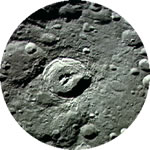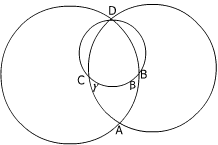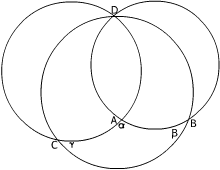#### You may also like### Baby Circle

A small circle fits between two touching circles so that all three circles touch each other and have a common tangent? What is the exact radius of the smallest circle?### Kissing

Two perpendicular lines are tangential to two identical circles that touch. What is the largest circle that can be placed in between the two lines and the two circles and how would you construct it?### Logosquares

Ten squares form regular rings either with adjacent or opposite vertices touching. Calculate the inner and outer radii of the rings that surround the squares.

# Lunar Angles

##### Age 16 to 18Challenge LevelThe angle between two circles at the point of intersection is the angle between the tangents to the circles at that point contained in the overlapping area or lune. Prove that, for any two circles, the angles at both points of intersection are equal.

Three circles intersect at the point $D$ and, in pairs, at the points $A,\ B$ and $C$ so that the arcs $AB$, $BC$ and $CA$ form a curvilinear triangle with interior angles $\alpha$, $\beta$ and $\gamma$ respectively. The diagrams show two possible cases. Prove that, for any three such circles, $\alpha + \beta + \gamma = \pi$.When you have done this question you might like to consider what happens on the surface of a sphere where instead of a flat surface (Euclidean geometry) you are working on a surface with positive curvature (Spherical or Elliptic geometry). See the article

'Where the angles of a triangle don't add up to 180 degrees'.

On the surface of a sphere the straight lines (lines of shortest distance between points) are great circles, the angles in a triangle add up to more than $\pi$ and the area of a triangle depends on its angles not on the lengths of the sides.